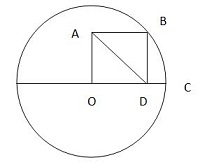Diagonal Length

Geometry Level 1

In the figure below, O is the center of the circle with radius 2 and vertex of the square OABC. What is the length of AD?×